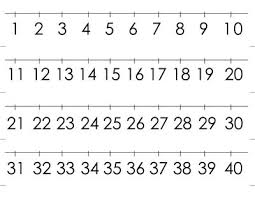# Modifications 7479

The Numerometer has invented the number machine that changes numbers until it makes them single-digit numbers. He still makes the change according to the same rule. For example: from the number 87312, after six modifications, he gradually made the number 3 as follows: 87312 → 15312 → 6312 → 912 → 102 → 12 → 3
a) Write the following two numbers, which will make from number 24681.
b) To which one-digit number will the number 987654321 change after modifications?
c) How many adjustments does the machine need to change a 100-digit number written in nines to a 50-digit number written in nines only?

a1 =  6681
a2 =  1281
b =  9
c =  100

### Step-by-step explanation:Did you find an error or inaccuracy? Feel free to write us. Thank you!

Tips for related online calculators